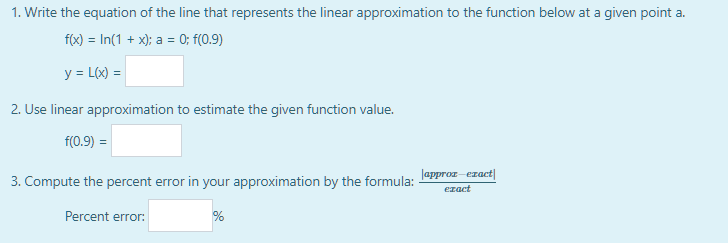3 questions

reopened

f(a)=ln(1+a); df/dx=1/(1+x)=1/(1+a) at point (a,ln(1+a)) and is the slope of the linear approximation.

When a=0, f(a)=ln(1)=0 and the point is (0,0).

L(x)-ln(1+a)=(1/(1+a))(x-a). When a=0, L(x)=x.

f(0.9)=ln(1-0.1) that is, x=-0.1, so L(-0.1)=-0.1 approximating to f(-0.1)=ln(1-0.1)=ln(0.9).

Actual ln(0.9)=-0.10536...

Percent error = |-0.1+0.10536|/-0.10536=-0.00536/0.10536=-0.0509 approx, that is 5.09% below the actual value so it is 94.91% accurate.

by Top Rated User (804k points)
selected by